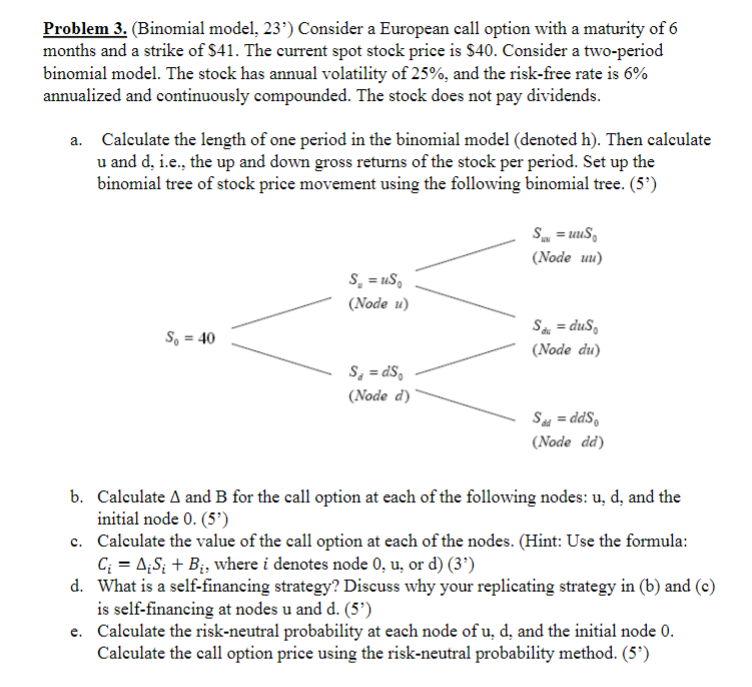Home / Expert Answers / Finance / problem-3-binomial-model-23-39-consider-a-european-call-option-with-a-maturity-of-6-months-and-a-pa242

# (Solved): Problem 3. (Binomial model, 23') Consider a European call option with a maturity of 6 months and a ...Problem 3. (Binomial model, 23') Consider a European call option with a maturity of 6 months and a strike of \$41. The current spot stock price is \$40. Consider a two-period binomial model. The stock has annual volatility of , and the risk-free rate is annualized and continuously compounded. The stock does not pay dividends. a. Calculate the length of one period in the binomial model (denoted ). Then calculate and d, i.e., the up and down gross returns of the stock per period. Set up the binomial tree of stock price movement using the following binomial tree. (5') b. Calculate and for the call option at each of the following nodes: , and the initial node 0 . (5') c. Calculate the value of the call option at each of the nodes. (Hint: Use the formula: , where denotes node , or d) (3') d. What is a self-financing strategy? Discuss why your replicating strategy in (b) and (c) is self-financing at nodes and . (5') e. Calculate the risk-neutral probability at each node of , and the initial node 0 . Calculate the call option price using the risk-neutral probability method. (5')

We have an Answer from Expert